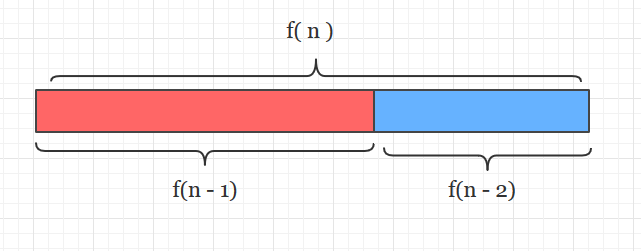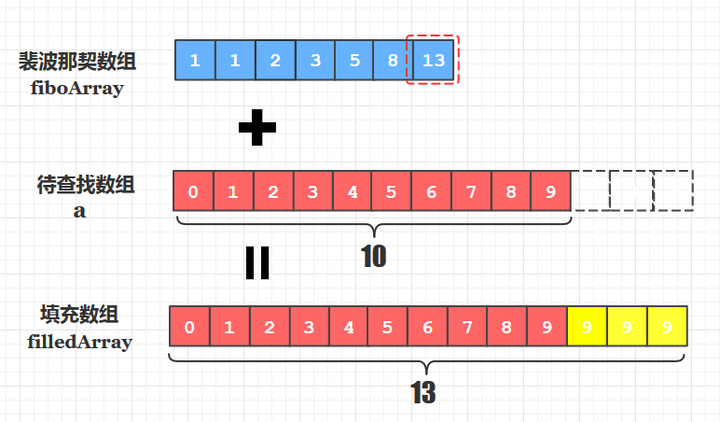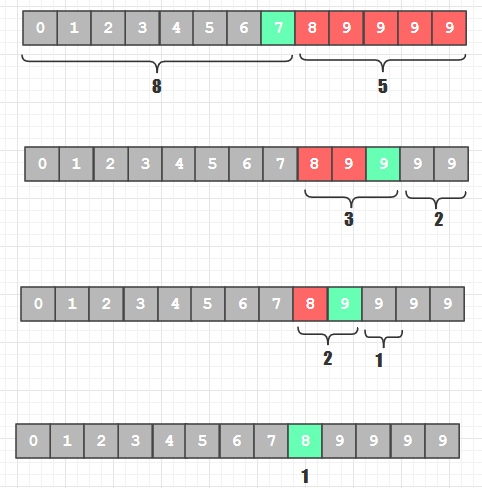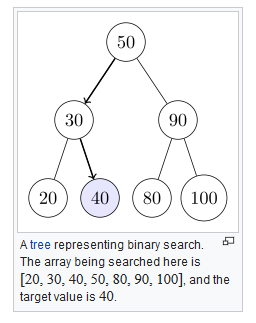# 裴波那契查找的来源

1、1、2、3、5、8、13、21 ... ...（注意一个细节： 在分割时，可以选择将“大块”的f(n-1)放前面部分，也可以将“小块”的f(n-2)放前面，我下面的分割都是按照“大块”在前进行的）

# 三个数组以及它们之间的关系。

• 待查找数组 (a)
• 裴波那契数组(fiboArray)
• 填充后数组(filledArray)# 查找到填充的部分元素如何处理？

## 查找过程

OK，有了上面的基础我们总结下查找的过程：

1. 根据待查找数组长度确定裴波那契数组的长度（或最大元素值）
2. 根据1中长度创建该长度的裴波那契数组，再通过F(0)=1，F(1)=1, F(n)=F(n-1)+F(n-2)生成裴波那契数列为数组赋值
3. 以2中的裴波那契数组的最大值为长度创建填充数组，将原待排序数组元素拷贝到填充数组中来， 如果有剩余的未赋值元素， 用原待排序数组的最后一个元素值填充
4. 针对填充数组进行关键字查找， 查找成功后记得判断该元素是否来源于后来填充的那部分元素

package find;import java.util.Scanner;public class FibonacciSearch {    public static void main(String[] args) {        int[] a={0,1,2,3,4,5,6,7,8,9};        System.out.println("请输入想要查找的数值：");        Scanner sc=new Scanner(System.in);        int key=sc.nextInt();        int s=search(a,key);        if(s==-1){            System.out.println("没有这个数据");        }else{            System.out.println("查到数据下标为"+s);            System.out.println("查到数据为第"+(s+1)+"个数");        }    }    /**     * @param a: 待查找的数组     * @description: 创建最大值刚好>=待查找数组长度的裴波纳契数组     */    private static int[] makeFiboArray(int[] a) {        int N = a.length;        int first = 1, sec = 1, third = 2, fbLength = 2;        int higt = a[N - 1];        while (third < N) {        // 使得裴波那契数不断递增，直到值刚好大于等于原数组长度为止            third = first + sec;   // 根据f(n) = f(n-1)+ f(n-2)计算            first = sec;            sec = third;            fbLength++;// 计算最后得到的裴波那契数组的长度        }        int[] fb = new int[fbLength];  // 根据上面计算的长度创建一个空数组        fb = 1;    // 第一和一二个数是迭代计算裴波那契数的基础        fb = 1;        for (int i = 2; i < fbLength; i++) {            fb[i] = fb[i - 1] + fb[i - 2];  // 将计算出的裴波那契数依次放入上面的空数组中        }        return fb;    }    /**     * @description: 裴波那契查找     */    public static int search(int[] a, int key) {        int low, high;        int lastA;        int[] fiboArray = makeFiboArray(a);//// 创建最大值刚好>=待查找数组长度的裴波纳契数组        int filledLength = fiboArray[fiboArray.length - 1];//创建填充数组长度        int[] filledArray = new int[filledLength];// 创建长度等于裴波那契数组最大值的填充数组        for (int i = 0; i < a.length; i++) {            filledArray[i] = a[i];// 将原待排序数组的元素都放入填充数组中        }        lastA = a[a.length - 1];//// 原待排序数组的最后一个值        for (int i = a.length; i < filledLength; i++) {            filledArray[i] = lastA;//// 如果填充数组还有空的元素，用原数组最后一个元素值填满        }        low = 0;        high = a.length; // 取得原待排序数组的长度 （注意是原数组！）        int mid;        int k = fiboArray.length - 1;        while (low <= high) {            mid = low + fiboArray[k - 1] - 1;            if (key < filledArray[mid]) {                high = mid - 1;//排除右半边的元素                k = k - 1;//f(k-1)是左半边的长度            } else if (key > filledArray[mid]) {                low = mid - 1;//排除左半边的元素                k = k - 2;//f(k-2)是右半边的长度            } else {                if (mid > high) {//说明取得了填充数组末尾的重复元素了                    return high;                } else {                    return mid;//说明没有取到填充数组末尾的重复元素                }            }        }        return -1;    }}• 我上面介绍的版本： 先把斐波那契数算出来，再全部用数组存起来， 要用的时候直接从数组里拿就可以了
• 这个版本： 不用数组存， 只算出来需要的最大的斐波那契数， 要用的时候“临时”计算就可以了

## 二分，插值和裴波纳契查找的性能比较posted @ 2020-07-10 14:51  Amber-  阅读(164)  评论(0编辑  收藏